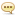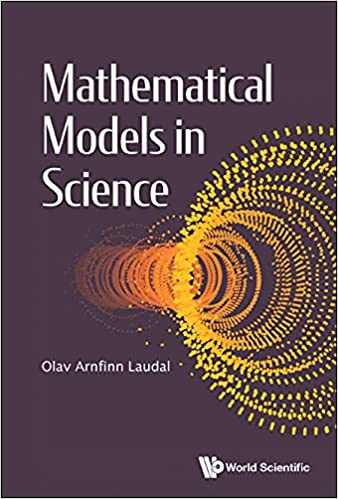eBooks :

# Mathematical Models In Science

Author: Baturi   |   18 September 2021   |: 0English | 2021 | ISBN: 1800610270 | 319 pages | True PDF EPUB | 30.49 MB
Mathematical Models in Science treats General Relativity and Quantum Mechanics in a non-commutative Algebraic Geometric framework.Based on ideas first published in Geometry of Time-Spaces: Non-commutative Algebraic Geometry Applied to Quantum Theory (World Scientific, 2011), Olav Arnfinn Laudal proposes a Toy Model as a Theory of Everything, starting with the notion of the Big Bang in Cosmology, modeled as the non-commutative deformation of a thick point. From this point, the author shows how to extract reasonable models for both General Relativity and Quantum Theory. This book concludes that the universe turns out to be the 6-dimensional Hilbert scheme of pairs of points in affine 3-space. With this in place, one may develop within the model much of the physics known to the reader. In particular, this theory is applicable to the concept of Dark Matter and its effects on our visual universe.Hence, Mathematical Models in Science proves the dependency of deformation theory in Mathematical Physics and summarizes the development of physical applications of pure mathematics developed in the twentieth century.